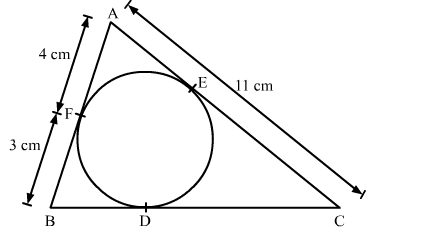# In the given figure, the length of BC is`
Question:

In the given figure, the length of BC is

(a) 7 cm
(b) 10 cm
(c) 14 cm
(d) 15 cmSolution:

We know that tangent segments to a circle from the same external point are congruent.
Therefore, we have
AF = AE = 4 cm
BF = BD = 3 cm
EC = AC − AE = 11 −  4 = 7 cm
CD = CE = 7 cm
∴ BC = BD + DC = 3 + 7 = 10 cm
Hence, the correct answer is option (b).